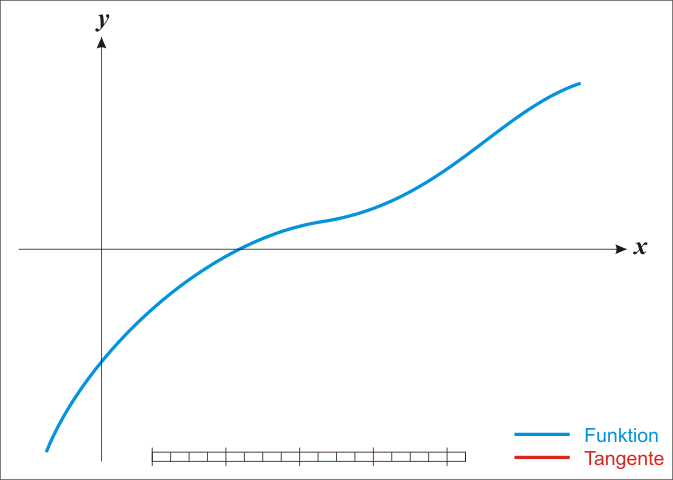Vect tip of the week : Newton’s method

Hi all and welcome to another vect tip of the week.

So, while doing a homework for a numerical solution of partial differential equations class (or NSoPDE, as I call it), I thought about if I could write the Newton’s method in Matlab so that I could make it faster. But first, let’s do a little recap of what Newton’s method is.

Think about your favorite non-linear equation (because linear equations are too mainstream :P). For the sake of this post, we shall make do with$sin(x)=0$, which as everybody should know, has solutions at$x=k\pi, k\in\mathbb{Z}$.

Let us now suppose that we don’t know anything about this function! The only thing we have is a black box that takes a number x and gives back a number y, the value of the sin function at x. Thus, we can’t use algebra to get our roots (which is of course what we want if we are to simulate what usually happens and why we need a method such as Newton’s).

The idea is this. If we have a non-linear function f(x) for which we want to find the roots of the equation f(x)=0, we can first pick a point$x_{0}$. Supposing that f is at least one time differentiable and$x_{0}$ is not a root of the derivative, we can continue. We take the tangent at that point, which comes from the equation :$y=f(x_{0})+f'(x_{0})(x-x_{0})\Rightarrow x-x_{0}-\frac{y-f(x_{0})}{f'(x_{0})}=0$

And guess what? This tangent is a good approximation of f when we are talking about x close to$x_{0}$ (How close? Think taylor expansion). Now, if we use this idea and solve for x, we get$x_{1}=x=x_{0}+\frac{f(x)-f(x_{0})}{f'(x_{0})}=x_{0}-\frac{f(x_{0})}{f'(x_{0})}$

where we used that we are talking the tangent passing from the points$\left(x_{0},f(x_{0})\right),\ \left(x_{1},0\right)$.

Long story short, we can use the new$x_{1}$ as a starting point again and calculate an$x_{2}$. And then repeat the process again and again, until we get a value we like. The method is illustrated in the following diagram.Implementing this in Matlab is easy and could be done naively like this,

function y = newton(f,fprime,x0)
maxIter = 100;
tol = 1e-7;
iter = 1;
error = 1;

while(error<tol && iter
temp = x0 - f(x0)/fprime(x0);
error = abs(f(x0));
x0 = temp;
iter = iter + 1;
end
fprintf('The solution is %f\n',x0);
end


This (naive) implementation should do the trick. It just takes as input a function f, a starting point$x_{0}$ and it’s derivative fprime and calculates a new x until either the value of at that point is less that tol, which stands for tolerance, or until the variable iteration reaches the maximum number of iterations. That’s just protection against infinite loops. I know that you hate those as much as I do.

The only thing that this program does is to calculate the recursion$x_{n+1}=x_{n}-\frac{f(x_{n})}{f'(x_{n})}$

and thus you may wonder, what can be done to make the process go faster?

Well, when we are searching for one root, then all we can do is to work on the math side to find a suitable$x_{0}$ close to the real root. Or use a bracketing method like bisection to find that$x_{0}$ numerically and then let Newton’s do the rest. For these kind of problems, Newton’s is blazingly fast! 😀

Now let’s say that we have a function and we have to find multiple roots of it. The example of$sin(x)=0$ is a suitable toy problem because we know all the roots through periodicity. Of course that’s not always the case.

So, what can we do? We can use Newton’s to find multiple roots without having to call the function multiple times. Instead of a starting point, we have to give a vector of different starting points and also change the error variable to something more suitable.

function y = newton(f,fprime,x0)
%x0 can be a vector in this example
maxIter = 100;
tol = 1e-7;
iter = 1;
error = 1;

while(error<tol && iter
temp = x0 - f(x0)/fprime(x0);
error = max(abs(f(x0)));
x0 = temp;
iter = iter + 1;
end
fprintf('The solution is %f\n',x0);
end


Would something like this work? Probably so and you are free to try it out. It’s not too fancy, we just do Newton’s method for different starting points at the same time using the vectorization capabilities of Matlab.